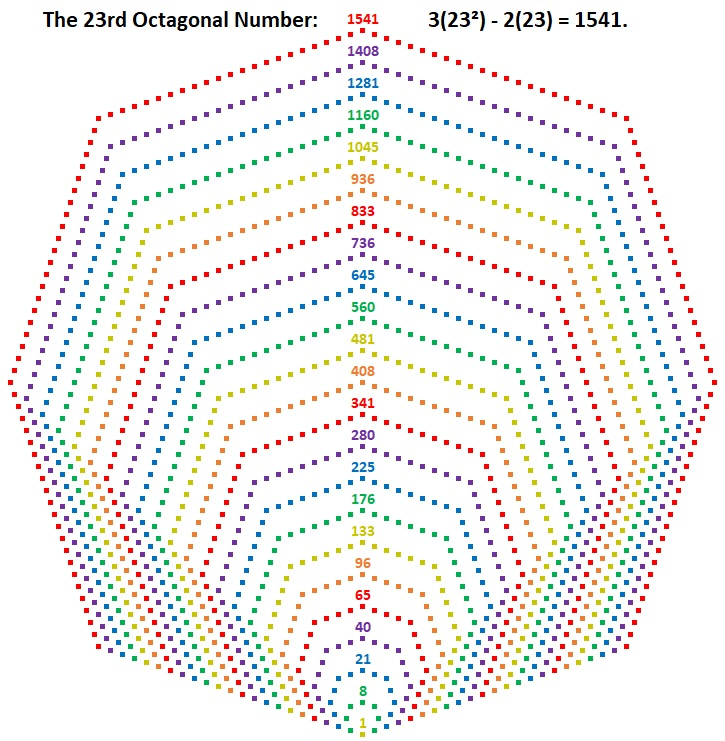# 1541 is the 23rd Octagonal Number

Contents

### Today’s Puzzle:

Into which geometric shape can you arrange 1541 tiny squares?

The answer is an octagon as illustrated below.Can you divide that octagon into 6 triangles, 5 of them representing the 22nd triangular number and 1 of them representing the 23rd?

It is possible because 5(22·23)/2 + (23·24)/2 = 1541.

We can simplify the left side of the equal sign:
5(22·23)/2 + (23·24)/2 =
5(11·23) + (23·12) =
23(5·11) + 23(12) =
23(55 + 12) =
23(67) = 1541

### Factors of 1541:

• 1541 is a composite number.
• Prime factorization: 1541 = 23 × 67.
• 1541 has no exponents greater than 1 in its prime factorization, so √1541 cannot be simplified.
• The exponents in the prime factorization are 1 and 1. Adding one to each exponent and multiplying we get (1 + 1)(1 + 1) = 2 × 2 = 4. Therefore 1541 has exactly 4 factors.
• The factors of 1541 are outlined with their factor pair partners in the graphic below.### Another Fact about the Number 1541:

1541 is the difference of two squares in two different ways:
771² – 770² = 1541 (Not coincidently, 770 + 771 = 1541), and
45² – 22² = 1541.

This site uses Akismet to reduce spam. Learn how your comment data is processed.